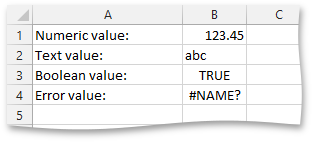20.2 (EAP/Beta)
20.1
19.2
19.1
18.2
18.1
17.2
.NET Framework 4.5.2+
.NET Framework 4.5.2+
.NET Standard 2.0+

# How to: Create a Worksheet Cell and Set Its Value

The example below demonstrates how to add cells to a worksheet. To do this, call the IXlRow.CreateCell method of the row where you wish to create a cell. If required, pass the following parameter: the zero-based index of the column where the new cell should be located. As a result, the cell will be created at the intersection of the specified row and column.

Each cell in a worksheet can contain a single piece of data - the cell value specified by the XlVariantValue object. To set a cell value, assign the required value to the IXlCell.Value property. A cell value can be of one of the following types: numeric, text, Boolean or error. Data values of these types can have various display formats. For example, a numeric value can be displayed as a decimal number, a percentage or currency value, a date or time value, etc. To specify the cell number format, use the IXlCell.Formatting property.

##### NOTE

A complete sample project is available at https://github.com/DevExpress-Examples/xl-export-api-examples-t253492

``````// Create a worksheet.
using (IXlSheet sheet = document.CreateSheet()) {
// Create the column A and set its width.
using (IXlColumn column = sheet.CreateColumn()) {
column.WidthInPixels = 150;
}

// Create the first row.
using (IXlRow row = sheet.CreateRow()) {

// Create the cell A1 and set its value.
using (IXlCell cell = row.CreateCell()) {
cell.Value = "Numeric value:";
}

// Create the cell B1 and assign the numeric value to it.
using (IXlCell cell = row.CreateCell()) {
cell.Value = 123.45;
}
}

// Create the second row.
using (IXlRow row = sheet.CreateRow()) {

// Create the cell A2 and set its value.
using (IXlCell cell = row.CreateCell()) {
cell.Value = "Text value:";
}

// Create the cell B2 and assign the text value to it.
using (IXlCell cell = row.CreateCell()) {
cell.Value = "abc";
}
}

// Create the third row.
using (IXlRow row = sheet.CreateRow()) {

// Create the cell A3 and set its value.
using (IXlCell cell = row.CreateCell()) {
cell.Value = "Boolean value:";
}

// Create the cell B3 and assign the boolean value to it.
using (IXlCell cell = row.CreateCell()) {
cell.Value = true;
}
}

// Create the fourth row.
using (IXlRow row = sheet.CreateRow()) {

// Create the cell A4 and set its value.
using (IXlCell cell = row.CreateCell()) {
cell.Value = "Error value:";
}

// Create the cell B4 and assign an error value to it.
using (IXlCell cell = row.CreateCell()) {
cell.Value = XlVariantValue.ErrorName;
}
}
}
``````

The image below shows the result (the workbook is opened in Microsoft® Excel®).